# A tour of mrcal: cross-validation

## Cross-validation

We now have a good method to evaluate the quality of a calibration: the projection uncertainty. Is that enough? If we run a calibration and see a low projection uncertainty, can we assume that the computed model is good, and use it moving forward? Once again, unfortunately, we cannot. A low projection uncertainty tells us that we're not sensitive to noise in the observed chessboard corners. However it says nothing about the effects of model errors.

Anything that makes our model not fit produces a model error. These can be caused by any of (for instance)

• out-of focus images
• images with motion blur
• rolling shutter effects
• camera synchronization errors
• chessboard detector failures
• insufficiently-rich models (of the lens or of the chessboard shape or anything else)

If model errors were present, then

• the computed projection uncertainty would underestimate the expected errors: the non-negligible model errors would be ignored
• the computed calibration would be biased: the residuals $$\vec x$$ would be heteroscedastic, so the computed optimum would not be a maximum-likelihood estimate of the true calibration (see the noise modeling page)

By definition, model errors are unmodeled, so we cannot do anything with them analytically. Instead we try hard to force these errors to zero, so that we can ignore them. To do that, we need the ability to detect the presense of model errors. The solve diagnostics we talked about earlier are a good start. An even more powerful technique is computing a cross-validation diff:

• We gather not one, but two sets of chessboard observations
• We compute two completely independent calibrations of these cameras using the two independent sets of observations
• We use the mrcal-show-projection-diff tool to compute the difference.

The two separate calibrations sample the input noise and the model noise. This is, in effect, an empirical measure of uncertainty. If we gathered lots and lots of calibration datasets (many more than just two), the resulting empirical distribution of projections would conclusively tell us about the calibration quality. Here we try to get away with just two empirical samples and the computed projection uncertainty to quantify the response to input noise.

If the model noise is negligible, as we would like it to be, then the cross-validation diff contains sampling noise only, and the computed uncertainty becomes the authoritative gauge of calibration quality. In this case we would see a difference on the order of $$\mathrm{difference} \approx \mathrm{uncertainty}_0 + \mathrm{uncertainty}_1$$. It would be good to define this more rigorously, but in my experience, even this loose definition is sufficient, and this technique works quite well.

For the Downtown LA dataset I did gather more than one set of images, so let's compute the cross-validation diff using our data.

We already saw evidence that LENSMODEL_OPENCV8 doesn't fit well. What does its cross-validation diff look like?

mrcal-show-projection-diff           \
--cbmax 2                          \
--unset key                        \
2-f22-infinity.opencv8.cameramodel \
3-f22-infinity.opencv8.cameramodel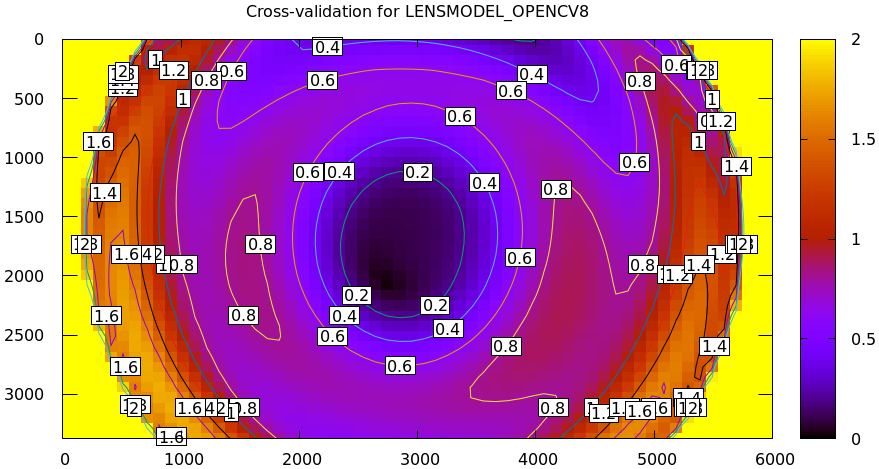A reminder, the computed dance-2 uncertainty (response to sampling error) looks like this: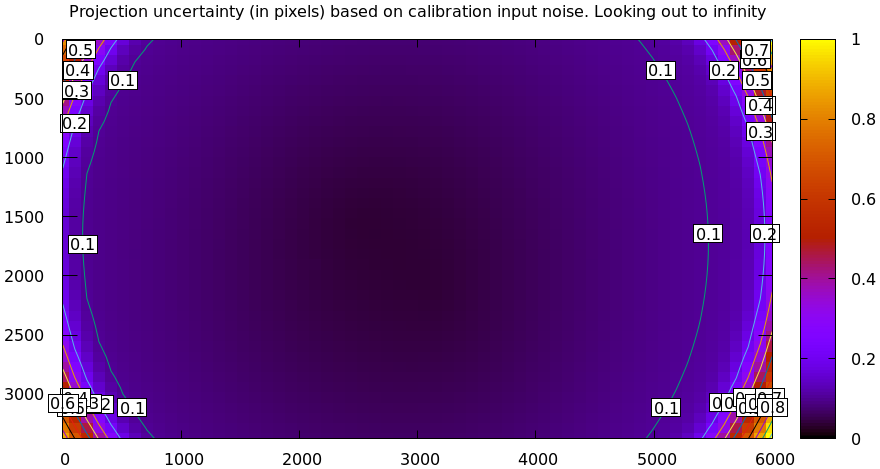The dance-3 uncertainty looks similar. So if we have low model errors, the cross-validation diff whould be within ~0.2 pixels in most of the image. Clearly this model does far worse than that. So we can conclude that LENSMODEL_OPENCV8 doesn't fit well.

We expect the splined model to do better. Let's see. The cross-validation diff:

mrcal-show-projection-diff           \
--cbmax 2                          \
--unset key                        \
2-f22-infinity.splined.cameramodel \
3-f22-infinity.splined.cameramodel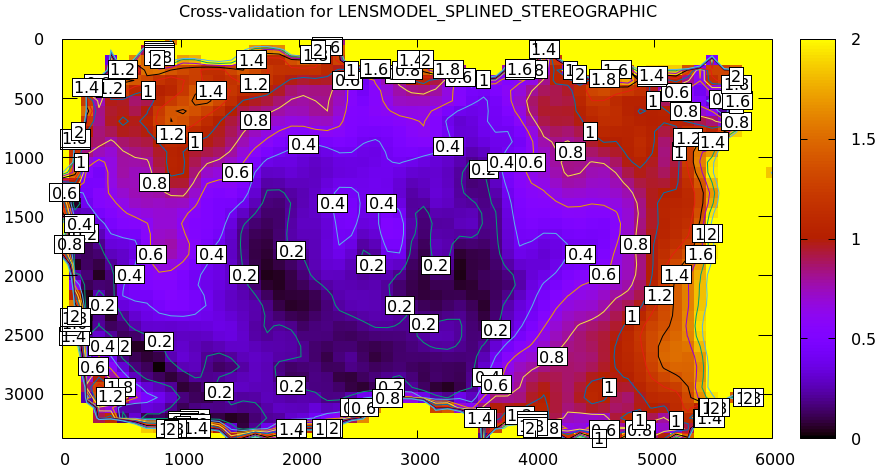And the dance-2 uncertainty (from before):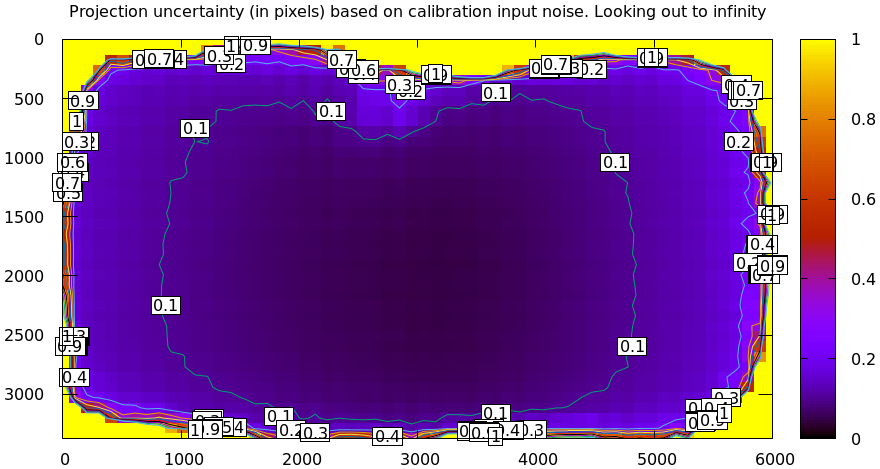Much better. It's an improvement over LENSMODEL_OPENCV8, but it's still noticeably not fitting. So we can explain ~0.2-0.4 pixels of the error away from the edges (twice the uncertainty), but the other 0.5-1.0 pixels of error (or more if considering the data at the edges) is unexplained. Thus any application that requires an accuracy of <1 pixel would have problems with this calibration.

I know from past experience with this lens that the biggest problem here is caused by mrcal assuming a central projection in its models: it assumes that all rays intersect at a single point. This is an assumption made by more or less every calibration tool, and most of the time it's reasonable. However, this assumption breaks down when you have a physically large, wide-angle lens looking at objects very close to the lens: exactly the case we have here.

In most cases, you will never use the camera system to observe extreme closeups, so it is reasonable to assume that the projection is central. But this assumption breaks down if you gather calibration images so close as to need the noncentral behavior. If the calibration images were gathered from too close, we would see a too-high cross-validation diff, as we have here. The recommended remedy is to gather new calibration data from further out, to minimize the noncentral effects. The current calibration images were gathered from very close-in to maximize the projection uncertainty. So getting images from further out would produce a higher-uncertainty calibration, and we would need to capture a larger number of chessboard observations to compensate.

Here I did not gather new calibration data, so we do the only thing we can: we model the noncentral behavior. A branch of mrcal contains an experimental and not-entirely-complete support for noncentral projections. I solved this calibration problem with that code, and the result does fit our data much better. The cross-validation diff: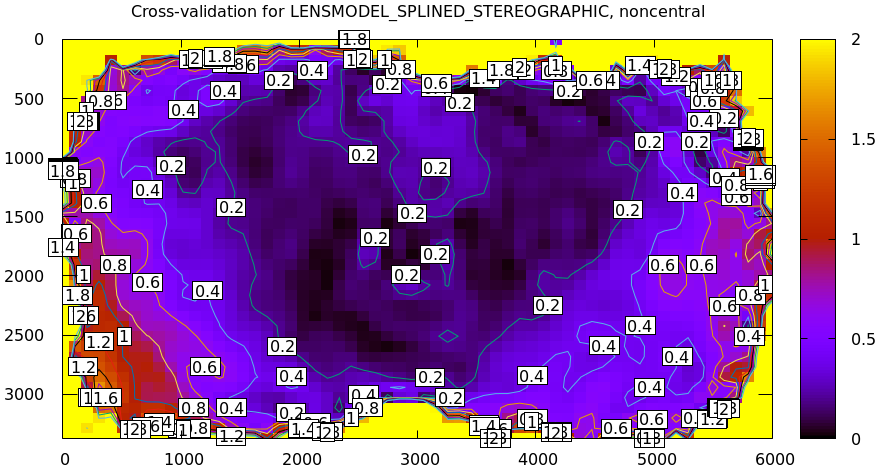This still isn't perfect, but it's close. The noncentral projection support is not yet done. Talk to me if you need it.

A more rigorous interpretation of these cross-validation results would be good, but a human interpretation is working well, so it's low-priority for me at the moment.